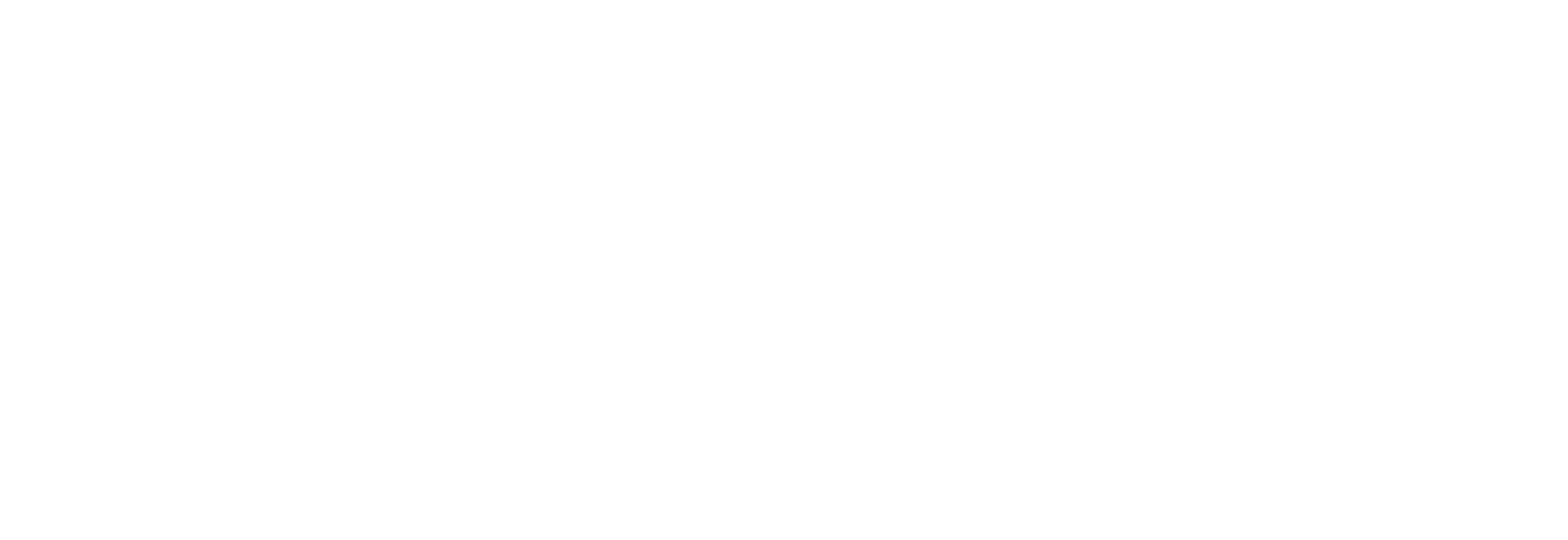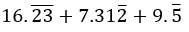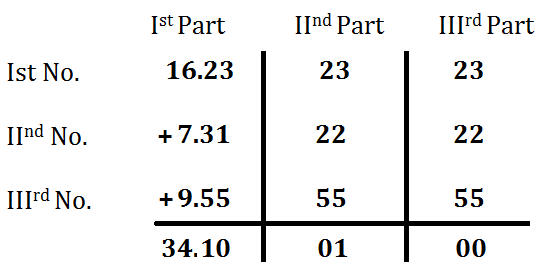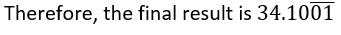Question of The Day22-06-2020

What will be the value of $$16.\bar{23} + 7.31\bar{2} + 9.\bar{5}$$?

Correct Answer : d )Explanation :

Consider the given expressionTo add or subtract the recurring numbers we should do the following steps

First divide all the number into three parts such that first part of all the numbers will contain same number of digits and second part of all the numbers contain same number of digits.

Part 1: First part will have all the non-recurring digits from all the numbers (If all the numbers do not have equal number of digits after decimal, then take some part of recurring digits to make them equal)

Here, first part of first number = 16.23

First part of second number = 7.31

First part of third number = 9.55

Part 2: Second part will comprise of remaining numbers left after taking the first part from the numbers. Second part will contain digits equal to LCM of Number of Recurring digits in all the numbers.

Here, number of recurring digits in all the number are 23, 2 and 5

So, number of digits in second part = LCM (2, 1, and 1) = 2

Thus, second part of first number = 23

Second part of second number = 22

Second part of third number = 55

Part 3: Take only two digits from the digits remaining after taking first part and second part

So, third part of first number = 23

Second part of second number = 22

Second part of third number = 55

Then, we will do the addition or subtraction as normal addition or subtraction and make the values of second part as recurring digits and leave the third part.Hence, (d) is the correct answer.

Such type of question is asked in various government exams like SSC CGL, SSC MTS, SSC CPO, SSC CHSL, RRB JE, RRB NTPC, RRB GROUP D, RRB OFFICER SCALE-I, IBPS PO, IBPS SO, RRB Office Assistant, IBPS Clerk, RBI Assistant, IBPS RRB OFFICER SCALE 2&3, UPSC CDS, UPSC NDA, etc.

Read Daily Current Affairs, Banking Awareness, Hindi Current Affairs, Word of the Day, and attempt free mock tests at PendulumEdu and boost your preparation for the actual exam.0